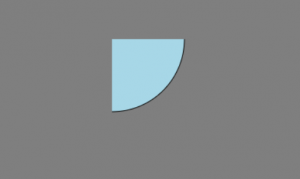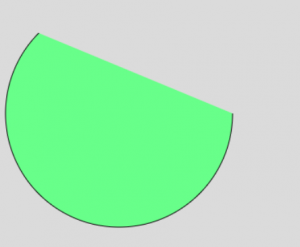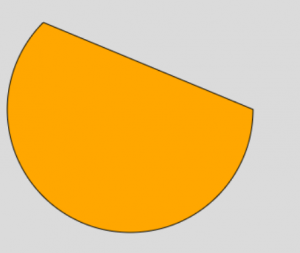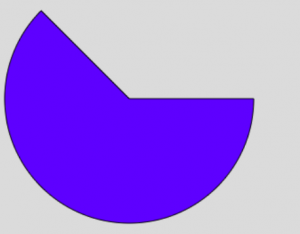Related Articles

# p5.js | arc() Function

• Difficulty Level : Medium
• Last Updated : 24 Oct, 2018

The arc() function is an inbuilt function in p5.js which is used to draw an arc. This function accepts seven parameters which are x-ordinate, y-ordinate, width, height, start, stop and an optional parameter mode.

Syntax:

`arc(x, y, w, h, start, stop, mode)`

Parameters: This function accepts seven parameters as mentioned above and described below:

• x: This parameter is used to hold the value of x-coordinate of the arc of ellipse.
• y: This parameter is used to hold the value of y-coordinate of the arc of ellipse.
• w: This parameter takes the value of width of the arc of ellipse.
• h: This parameter takes the value of height of the arc of ellipse.
• start: This parameter takes the value of angle to start the arc, specified in radians.
• stop: This parameter takes the value of angle to stop the arc, specified in radians.
• mode: This is an optional parameter which determines the way of drawing the arc either CHORD, PIE or OPEN
• Program 1: This program uses the DEFAULT mode.
 `function` `setup() {``    ``createCanvas(400, 400);``}`` ` `function` `draw() {``    ``background(``'gray'``);`` ` `    ``// Quarter arc at 150, 55 of height and width 290px``    ``arc(150, 55, 290, 290, 0, HALF_PI); ``    ``fill(``'lightblue'``);``}`

Output:• Program 2: This program uses the OPEN mode.
 `function` `setup() {``    ``createCanvas(400, 400);``}`` ` `function` `draw() {``    ``background(220);``    ``fill(``'lightgreen'``);`` ` `    ``// An open arc at 150, 150 with radius 280``    ``arc(150, 150, 280, 280, 0, PI + QUARTER_PI, OPEN); ``}`

Output:• Program 3: This program uses the CHORD mode.
 `function` `setup() {``    ``createCanvas(400, 400);``}`` ` `function` `draw() {``    ``background(220);``    ``fill(``'orange'``);`` ` `    ``// A chord-arc at 150, 150 with radius 280``    ``arc(150, 150, 280, 280, 0, PI + QUARTER_PI, CHORD); ``} `

Output:• Program 4: This program uses PIE mode.
 `function` `setup() {``    ``createCanvas(400, 400);``}`` ` `function` `draw() {``    ``background(220);``    ``fill(``'blue'``);``     ` `    ``// A pie-arc at 150, 150 with radius 280``    ``arc(150, 150, 280, 280, 0, PI + QUARTER_PI, PIE); ``} `

Output:References: https://p5js.org/reference/#/p5/arc

Hey geek! The constant emerging technologies in the world of web development always keeps the excitement for this subject through the roof. But before you tackle the big projects, we suggest you start by learning the basics. Kickstart your web development journey by learning JS concepts with our JavaScript Course. Now at it’s lowest price ever!

My Personal Notes arrow_drop_up